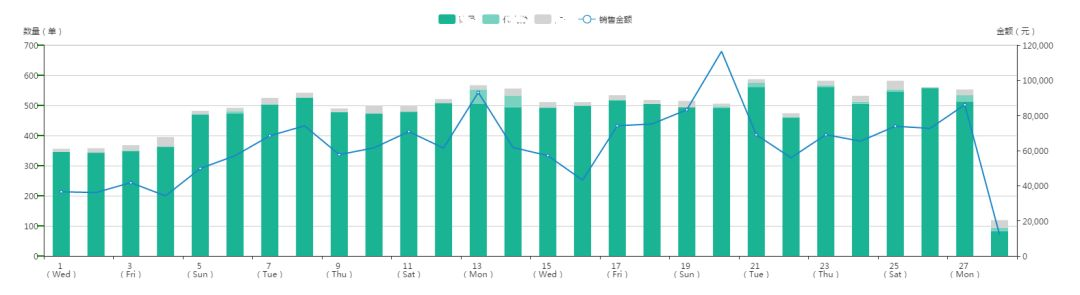# PHP+MySQL实现对一段时间内每天数据统计优化操作实例

http://www.jb51.net/article/136685.htmorder_id order_sn total_price enterdate
25396 A4E610E250C2D378D7EC94179E14617F 2306.00 2017-04-01 17:23:26

 `\$month` `= ` `'04'` `;` `\$year` `= ` `'2017'` `;` `\$max_day` `= cal_days_in_month(CAL_GREGORIAN, ` `\$month` `, ` `\$year` `);   ` `//当月最后一天` `//构造每天的数组` `\$days_arr` `= ` `array` `();` `for` `(` `\$i` `=1;` `\$i` `<=` `\$max_day` `;` `\$i` `++){` `  ` `array_push` `(` `\$days_arr` `, ` `\$i` `);` `}` `\$return` `= ` `array` `();` `//查询` `foreach` `(` `\$days_arr` `as` `\$val` `){` `  ` `\$min` `= ` `\$year` `.` `'-'` `.` `\$month` `.` `'-'` `.` `\$val` `.` `' 00:00:00'` `;` `  ` `\$max` `= ` `\$year` `.` `'-'` `.` `\$month` `.` `'-'` `.` `\$val` `.` `' 23:59:59'` `;` `  ` `\$sql` `= ` `"select count(*) as total_num,sum(`total_price`) as amount from `orders` where `enterdate` >= {\$min} and `enterdate` <= {\$max}"` `;` `  ` `\$return` `[] = mysqli_query(` `\$sql` `);` `}` `return` `\$return` `;`

 `\$month` `= ` `'04'` `;` `\$year` `= ` `'2017'` `;` `\$max_day` `= cal_days_in_month(CAL_GREGORIAN, ` `\$month` `, ` `\$year` `);   ` `//当月最后一天` `\$min` `= ` `\$year` `.` `'-'` `.` `\$month` `.` `'-01 00:00:00'` `;` `\$max` `= ` `\$year` `.` `'-'` `.` `\$month` `.` `'-'` `.` `\$max_day` `.` `' 23:59:59'` `;` `\$sql` `= ` `"select t.enterdate,count(*) as total_num,sum(t.total_price) as amount (select date_format(enterdate,'%e') as enterdate,total_price from orders where enterdate between {\$min} and {\$max}) t group by t.enterdate order by t.enterdate"` `;` `\$return` `= mysqli_query(` `\$sql` `);`

1.由于需查询当月所有数据，在数据量过大时，不宜采取本方法。

2.为避免当天没有数据而造成的数据缺失，在查询后，理应根据需求对数据进行处理。